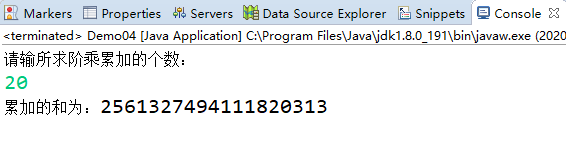• ## Java阶乘求和

千次阅读 2020-03-11 18:43:14
import java.util.Scanner; public class Demo { public static void main(String[] args) { Scanner input = new Scanner(System.in); System.out.println("请输所求阶乘累加的个数："); int...
题目：求1+2!+3!+...+20!的和 。

import java.util.Scanner;

public class Demo {
public static void main(String[] args) {
Scanner input = new Scanner(System.in);
System.out.println("请输所求阶乘累加的个数：");
int n = input.nextInt();
long test,sum = 0;

for(int i=1; i<=n; i++) {
test = 1;
for(int j=1; j<=i; j++) {
test *= j;
}
sum += test;
}
System.out.println("累加的和为：" + sum);
}
}

结果如下展开全文• Java计算阶乘，并对结果进行求和计算，具体是求1 2! 3! ... 20!的和，数学好的朋友，或许很轻松就编写出代码了，这就是一个比较简单的Java阶乘的代码例子，望参考。
• 在C语言中，对于累计的考察是十分频繁的，在选择、程序题中，总能见到累计问题的身影，比如最为简单的累计求和：1+2+3+4+....+100，再者斐波那契数列1+2+3+5+8+13+21+.......下面针对累计问题，系统的、从简到繁的带...
在C语言中，对于累计的考察是十分频繁的，在选择、程序题中，总能见到累计问题的身影，比如最为简单的累计求和：1+2+3+4+....+100，再者斐波那契数列1+2+3+5+8+13+21+.......下面针对累计问题，系统的、从简到繁的带着大家梳理一边首先，我们要明确，在程序中一看到sum=sum+i；s+=a；s+=a[i]等形式的式子，我们就要迅速联想到这题目是与累计问题相关的。例如：for(i=1;i<=10;i++)m=m+i;for(i=0;i<=9;i++){scanf(“%d”,a[i],sum=sum+a[i];}不难发现，设计累计问题的，都会有一个类似sum=sum+一个变量的式子，这就是我们解题的关键，甚至遇到此类题，我们可以先写上sum=sum+一个变量，然后去找具体的这个变量是什么就可以了。题型1：累计求和1+2+3+4+5+6+.....+100解析：首先写上sum=sum+i;(i是我们用来累加的一个变量)i是从1到100增加的，所以我们可能用for(i=1;i<=n;i++)来控制程序为int i,sum=0;for(i=1;i<=100;i++){ sum=sum+i; }题型二：正负交替问题1-2+3-4+5-6+.......+99-100解析：其实正负交替问题，可以看作是累计求和的一种，我们还是先写上sum=sum+i;在本题中，i不仅仅是从1到100增加的，还是正负交替变化的变化，那么我们就先实现从1到100增加，和上题一样，我们可能用for(i=1;i<=n;i++)，程序写到这里，就和上题是一样的：int i,sum=0;for(i=1;i<=n;i++){ sum=sum+i; }那正负交替如何实现呢，我们需要引用另外一个变量t=1，让t在循环体内实现正负的变化，即t=-t，所以最终程序为int i,sum=0,t=1;for(i=1;i<=100;i++){ sum=sum+i*t;t=-t;}题型三：斐波那契额数列求和1+2+3+5+8+13+21+34+.....求前20项之和解析：解法1：通过观察，可知，从第三项开始，每一项等于它的前两项之和，3=1+2，5=3+2，8=3+5。那么是累计问题，我们仍可先写上sum=sum+c，下面我们就来找这个c是什么从图片中，我们可以看出C的值就是我们要累计求和的数，c=a+b，所以可以写成int a=1,b=2,sum=3;for(i=3;i<=20;i++){c=a+b;sum=sum+c;a=b;b=c;}解法2：数组int arr = { 1, 2 },sum=0;int i = 0;for (i = 2; i < 20; i++)arr[i] = arr[i - 1] + arr[i - 2];for (i = 0; i < 20; i++)sum=sum+arr[i];printf("%d",sum);题型四：阶乘求1！+2！+3！+4！+......+10！解析：首先，我们还是写上sum=sum+一个数t，我们再来找这个数t，int i,t=1,sum=0;/*定义变量的数据类型为整型*/for(i=1;i<=10;i++)/*计算每个阶乘的值并累加*/{  t=t*i;/*计算每个阶乘的值*/sum=sum+t;}/*把每个阶乘的值累加*/printf("%d\n",sum);/*输出结果*/题型五：分子数列 2/1+3/2+5/3+8/5+......求前十项的和解析：我们还是先写上 sum=sum+t，然后来找这个t是什么根据题型3，我们可以看出，b/a正是我们要找的t.所以int a=1,b=2,c,i;float t,sum=0;for(i=1;i<=10;i++){t=1.0*b/a;sum=sum+t;c=a+b;a=b;b=c;}printf("%f",sum);#inclu题型六：输入5个学生的7门课程的成绩，每输入一个学生的7门课程成绩后，立即统计并输出该学生的int main(){ int i,j;float a,s;for(i=0;i<5;i++){s=0;for(j=0;j<7;j++){scanf("%f",&a[i][j]);s=s+a[i][j];}printf("第%d个学生的总分＝%.2f 平均分＝%.2f\n",i+1,s,s/7);}}春考有约，你与大学的约定
展开全文• 分段函数求解问题 判断一个整数是否为质数 求两个正整数的最大公约数 各种求和问题 3 掷骰子游戏的多种解法 打印规则的图形 穷举问题的多种求解方法 求阶乘 2010-122010-12-5 算法与语句 解决需要用分析推理、 解决...
本文由技术有点拉贡献ppt文档可能在WAP端浏览体验不佳。建议您优先选择TXT，或下载源文件到本机查看。高级语言程序设计12010-12-5第四章 程序流程控制主讲: 主讲: 计算机学院 朱立华22010-12-5内容提要算法的基本概念以及表示方法， 算法的基本概念以及表示方法，简单介绍流程图 的基本概念以及表示方法 程序的3种基本流程控制结构 顺序结构、 控制结构： C程序的3种基本流程控制结构：顺序结构、选择结构 也叫分支结构)、循环结构，每种结构的控制语句： )、循环结构 (也叫分支结构)、循环结构，每种结构的控制语句：if、switch控制选择结构 、 控制选择结构 ? for、while、do~while控制循环结构 、 、 控制循环结构 ? break、continue、goto等语句的用法 、 、 等 ? 循环嵌套程序的设计及运行过程一些常用算法的基本思想： 一些常用算法的基本思想：? ? ?分段函数求解问题 判断一个整数是否为质数 求两个正整数的最大公约数 各种求和问题3掷骰子游戏的多种解法 打印规则的图形 穷举问题的多种求解方法 求阶乘2010-122010-12-5算法与语句解决需要用分析推理、 解决需要用分析推理、逻 算法(Algorithm)就是为解决一个具体问题而采取 算法(Algorithm) 解决求数值解的问题 辑推理才能解决的问题的有限的操作步骤，算法通过语句来实现 的有限的操作步骤，算法通过语句来实现 语句 数据的描述和组织形式 对操作或行为的描述， 对操作或行为的描述， 计算机算法分两类：数值运算算法、 计算机算法分两类：数值运算算法、非数值运算算法 即操作步骤 程序=数据结构+ 程序=数据结构+算法 算法的正确性衡量标准： 算法的正确性衡量标准：有穷性：算法包含有限步操作 有穷性： ? 确定性：每一步都应确定无歧义 确定性： ? 有效性：每一步都应能有效执行且能得到确定的结果 有效性： ? 0或多个输入: 程序允许无输入 或多个输入: ? 1或多个输出：任何程序都必须有输出，哪怕是提示信息 或多个输出：任何程序都必须有输出，2010-122010-12-5 4算法与语句常用的算法描述方法有：自然语言、传统流程图、 常用的算法描述方法有：自然语言、传统流程图、NS 流程图、伪代码等，这里只介绍传统流程图。 流程图、伪代码等，这里只介绍传统流程图。 n!的算法思想 的算法思想： 例：求n!的算法思想： n!=1*2*3*… n!=1*2*3*…*n 由于计算机执行乘法时每次只能求两个数相乘， 由于计算机执行乘法时每次只能求两个数相乘，因此 上面的公式在程序中必定
展开全文• 求1到10的阶乘之和。/** * 1~10阶乘求和 */ public class Factorial { public static void main(String[] args) { int sum = 0; for (int i = 1; i &lt;= 10; i++) { sum += m1(i); ...
求1到10的阶乘之和。/**
* 1~10阶乘求和
*/
public class Factorial {
public static void main(String[] args) {
int sum = 0;
for (int i = 1; i <= 10; i++) {
sum += m1(i);
//sum += m2(i);

}
System.out.println(sum);
}

//求阶乘 方法一
private static int m1(int n) {
if (n == 1) return 1;
return n *= m1(n - 1);
}

//求阶乘 方法二
public static int m2(int n) {
if (n == 1) {
return 1;
}
int i = 1;
int res = 1;
while (i <= n) {
res *= i;
i++;
}
return res;
}
}
展开全文• publicclassMethodNestDemo{//求阶层之和publicstaticvoidmain(String[]args){MethodNestDemomnd=newMethodNestDemo();intn=10;//任意一个正整数intsum=0;for(inti=1;i<=n;i++){sum+=mnd.fact(i);...
• Java大数的阶乘和 import java.math.*; import java.util.Scanner; public class 大数的阶乘和 { public static void main(String[] args) { Scanner sc = new Scanner(System.in); System.out.print("请输入...
• 本文内容：使用java语言实现阶乘求和 实现思想： 阶乘求和主要用到循环； 先实现求阶乘的方法，再实现求和的方法，求和是调用求阶乘的方法 实现代码 public class practice { public static void main(String[] ...编程语言
• } java： import java.*; import java.awt.geom.AffineTransform; import java.math.BigInteger; import java.util.Scanner; public class Main{ public static void main(String []args) { Scanner cin = new ...
• 你知道，如何用递归实现连续整数求和阶乘吗?很多人表示对于具体的实现方式不是很清楚，下面就一起通过简单的例子来了解一下吧。packagecom.kite.test.recursion;publicclassRecursion{publicstaticvoidmain(String...
• java2实用教程书上找的基础题，一天一题，今天的题目是关于阶乘求和的。题目如下：编写应用程序求：1!+2!+3!+4!+5!+6!+7!+8!+9!+10!。求解这道题目的思路是，使用for循环计算从1到10每一个数字的阶乘的结果，然后...
• 题目描述 用高精度计算出S=1!...”表示阶乘，例如：5!=5×4×3×2×15!=5 \times 4 \times 3 \times 2 \times 15!=5×4×3×2×1。 输入输出格式 输入格式： 一个正整数NNN。 输出格式 ...
• ## Java作业-阶乘求和

万次阅读 2017-09-08 13:53:49
目标效果： 源码： /** * 求1+2!+3!+...+20!的和 * @author Vivinia * */ public class factorial { public static void main(String args[]) { ... //sum用于加和，num作为每一个数阶乘后的结果 for(ieclipse
• java 20的阶乘 求和 (注意 sum 是 int类型) public static void main(String[] args) { int sum=0; int num=1; for (int i = 1; i <=20 ; i++) { num=1; for (int k = 1; k <=i ; k++) { num*=k;算法
• 共回答了11个问题采纳率：100%1你的程序有很多的错误给你程序做了一下更改一个文件里只能有一个class,你的那个class Jiecheng{int show(int i){if(i==1)return 1;elsereturn i*show(i-1);}应该是个方法,不应该这么写...
• import java.util.Scanner; public class SentenceStructure { public static void main(String[] args) { System.out.println("请输入一个数字,表示 1!+2!+3!+…… 加到几："); Scanner scan = new Scanner...
• 题目 1014: [编程入门]阶乘求和 题目描述 求Sn=1!+2!+3!+4!+5!+…+n!之值，其中n是一个数字(n不超过20)。 输入 n 输出 Sn的值 样例输入 5 样例输出 153 import java.util.Scanner; public class Test12 { public ...
• 运用do-while编写10以内的阶乘求和 运用数学表达式就为：1!+2!+3!+4!+5!+6!+7!+8!+9!+10!=? 代码如下：import java.util.Scanner; public class factorial_10{ public static void main(String[] rags){ int num...string class
• ## Java阶乘的倒数求和

千次阅读 2014-03-18 08:53:21
public static void main(String[] args) { int sum = 1; double result = 0; Scanner sc = new Scanner(System.in);... System.out.println("请输入... System.out.println("阶乘倒数求和" + result); } }string
• import java.awt.geom.AffineTransform; import java.math.BigInteger; import java.util.Scanner; public class Main{ public static void main(String []args) { Scanner cin = new Scanner(System.in); int ...
• 1.使用公式C=（5/9） （ F-32）打印下列华氏温度与摄氏温度对照表： ...题目分析：两层循环的嵌套，第一层是所需要计算的所有数字，第二层是第一层中所有数字需要完成的阶乘计算，完成后求和并打印输出。
• ` Javapublic static void main(String[] args) {Scanner sc = new Scanner(System.in);int n = sc.nextInt();long sum = 0;for(int i = 1;i <= n;i++) {// 递归实现sum+=fun(i);}System.out.println(sum);}// ...
• import java.util.Scanner; public class _1013 { public static void main(String[] args) { Scanner scan = new Scanner(System.in); int n = scan.nextInt(); int a = 0; long sum = 0L; for(int i =...
• 第一个for循环是求和第二个是求阶乘这样看更易理解class jie{private static int ji(int i){int ji=1;for(int j =1;j<=i;j++)ji*=j;return ji;}public static void main(String args[]){long sum = 0;int i;for(i...
• // 递归求阶乘 static long recursion(int num) { if (num == 0) { return 0; } if (num == 1) { return 1; } return num * recursion(num - 1); }bash...

# java阶乘求和java 订阅